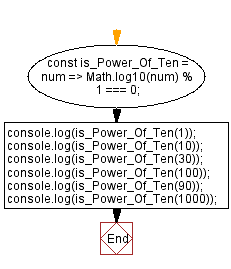# JavaScript: Check if a given number is a power of 10

## JavaScript Math: Exercise-54 with Solution

Write a JavaScript program to check if a given number is a power of 10.
Math.log10() and the modulo operator (%) determines if num is a power of 10.

Sample Solution:

HTML Code:

``````<!DOCTYPE html>
<html>
<meta charset="utf-8">
<title>JavaScript function to check if a  given number is a power of 10</title>
<body>

</body>
</html>
```
```

JavaScript Code:

``````const is_Power_Of_Ten = num => Math.log10(num) % 1 === 0;
console.log(is_Power_Of_Ten(1));
console.log(is_Power_Of_Ten(10));
console.log(is_Power_Of_Ten(30));
console.log(is_Power_Of_Ten(100));
console.log(is_Power_Of_Ten(90));
console.log(is_Power_Of_Ten(1000));
```
```

Sample Output:

```true
true
false
true
false
true
```

Flowchart:Live Demo:

See the Pen javascript-math-exercise-54 by w3resource (@w3resource) on CodePen.

Improve this sample solution and post your code through Disqus

Previous: Divide two complex numbers.
Next: Swap two variables

What is the difficulty level of this exercise?

Test your Programming skills with w3resource's quiz.

﻿